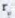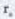Chapter 10, Problem 21SPFundamentals of Financial Manageme...

15th Edition
Eugene F. Brigham + 1 other
ISBN: 9781337395250

Solutions

Chapter
SectionFundamentals of Financial Manageme...

15th Edition
Eugene F. Brigham + 1 other
ISBN: 9781337395250
Textbook Problem

CALCULATING THE WACC Here is the condensed 2018 balance sheet for Skye Computer Company (in thousands of dollars):   2018 Current assets $2,000 Net fixed assets 3,000 Total assets$5,000 Accounts payable and accruals $900 Short-term debt 100 Long-term debt 1,100 Preferred stock (10,000 shares) 250 Common stock (50.000 shares) 1.300 Retained earnings 1,35O Total common equity$2,650 Total liabilities and equity $5,000 Skye's earnings per share last year were$3.20. The common stock sells for $55.00, last year’s dividend (D0) was$2.10, and a flotation cost of 10% would be required to sell new common stock. Security analysts are projecting that the common dividend will grow at an annual rate of 9%. Skye's preferred stock pays a dividend of $3.30 per share, and its preferred stock sells for$30.00 per share. The firm’s before-tax cost of debt is 10%, and its marginal tax rate is 35%. The firm's currently outstanding 10% annual coupon rate, long-term debt sells at par value. The market risk premium is 5%, the risk-free rate is 6%, and Skye's beta is 1.516. The firm s total debt, which is the sum of the company's short-term debt and long-term debt, equals $1.2 million. a. Calculate the cost of each capital component, that is, the after-tax cost of debt, the cost of preferred stock, the cost of equity from retained earnings, and the cost of newly issued common stock. Use the DCF method to find the cost of common equity. b. Now calculate the cost of common equity from retained earnings, using the CAPM method. c. What is the cost of new common stock based on the CAPM? (Hint Find the difference betweenandas determined by the DCF method, and add that differential to the CAPM value for.) d. If Skye continues to use the same market-value capital structure, what is the firm's WACC assuming that (1) it uses only retained earnings for equity and (2) if it expands so rapidly that it must issue new common stock? a. Summary Introduction To Determine: The after-tax cost of debt, cost of preferred stock, cost of common equity from retained earnings and cost of common equity from new common stock of Company SC. Introduction: WACC is abbreviated as weighted average cost of capital, is a equation that computes the average rate of return that an organization requires to acquire to repay its security holders or investors. This computation is utilized to determine if a project is beneficial or in the event that it just repays the expense of subsidizing the project. Explanation Determine the after-tax cost of debt AftertaxCostofDebt=[CostofDebt(rd)×(1TaxRate(T))]=[10%×(135%)]=[10%×65%]=6.50% Therefore the after-tax cost of debt is 6.50%. Determine the cost of preferred stock CostofPreferredStock(rP)=[PreferredDividends(DP)CostofPreferredStock]=[$3.30$30]=11% Therefore the cost of preferred stock is 11%. Determine the cost of common equity from retained earnings CostofRetainedEquity(rs)=[(D0(1+g)(P0))+g]=[($2

b.

Summary Introduction

To Determine: The cost of common equity from retained earnings of Company SC utilising CAPM method.

c.

Summary Introduction

To Determine: The cost of new common equity based on CAPM.

d.

Summary Introduction

To Determine: The WACC if the firm utilises only retained earnings for equity and if the firm expands rapidly in order to issue new common stock.

Still sussing out bartleby?

Check out a sample textbook solution.

See a sample solution

The Solution to Your Study Problems

Bartleby provides explanations to thousands of textbook problems written by our experts, many with advanced degrees!

Get Started

Find more solutions based on key concepts